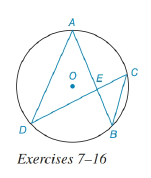Chapter 6.3, Problem 16EElementary Geometry For College St...

7th Edition
Alexander + 2 others
ISBN: 9781337614085

Solutions

Chapter
SectionElementary Geometry For College St...

7th Edition
Alexander + 2 others
ISBN: 9781337614085
Textbook Problem

In the figure for Exercises 7 to 16, O is the center of the circle. See Theorem 6.3.5.Given: A E = 6     a n d     E B = 4 ; D E : E C = 3 : 1 Find: DE and EC.To determine

To find:

To find DE and EC.

Explanation

Given that, AE=6andEB=4;DE:EC=3:1

The diagrammatic representation is given below,

Theorem:

If two chords intersect within a circle, then the product of the lengths of the segments (parts) of one chord is equal to the product of the lengths of the segments of the other chord.

By using the theorem to get the following,

AEEB=DEEC

Given that DE:EC=3:1 that is DE=3xandEC=x

Substitute the values AE=6andEB=4;DE=3xandEC=x in the above equation to get the following,

Still sussing out bartleby?

Check out a sample textbook solution.

See a sample solution

The Solution to Your Study Problems

Bartleby provides explanations to thousands of textbook problems written by our experts, many with advanced degrees!

Get Started

In Exercises 107-120, factor each expression completely. 113. 3x2 6x 24

Applied Calculus for the Managerial, Life, and Social Sciences: A Brief Approach

Simplify: 1218

Elementary Technical Mathematics

Sketch the graphs of the equations in Exercises 512. x2y=1

Finite Mathematics and Applied Calculus (MindTap Course List)

If f(x) = x2/(l + x), find f"(1).

Single Variable Calculus: Early Transcendentals, Volume I

Rewrite 10x2xdx using a trigonometric substitution. a) cos10sind b) 110sincos2d c) 10cos2sind d) 10sincosd

Study Guide for Stewart's Single Variable Calculus: Early Transcendentals, 8th